Filters Date Range Past year (0) Past month (0) Past week (0) Past day (0) Custom range... To   Search Extensions Types Contacts Categories Tag Types Tag Schemes View Results as: JSON ATOM CSV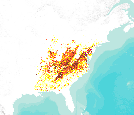This dataset represents actual and predicted suitable habitat for Liriodendron tuliperfia (yellow-poplar, species code 621) in the Eastern United States as measured by importance value based on data obtained from the Forest Inventory and Analysis (FIA) project, current climate conditions, and future climate projections. This summary unit of this dataset is a 20 by 20 kilometer cell. The actual importance value (IV) was calculated based on the number of stems and basal area of a given tree species relative to other tree species on a plot using about 100,000 FIA plots (representing nearly 3 million tree records) in the 37 states within the United States east of the 100th meridian. These importance values were summarized...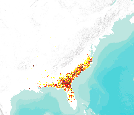This dataset represents actual and predicted suitable habitat for Nyssa sylvatica var. biflora (swamp tupelo, species code 694) in the Eastern United States as measured by importance value based on data obtained from the Forest Inventory and Analysis (FIA) project, current climate conditions, and future climate projections. This summary unit of this dataset is a 20 by 20 kilometer cell. The actual importance value (IV) was calculated based on the number of stems and basal area of a given tree species relative to other tree species on a plot using about 100,000 FIA plots (representing nearly 3 million tree records) in the 37 states within the United States east of the 100th meridian. These importance values were...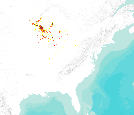This dataset represents actual and predicted suitable habitat for Quercus ellipsoidalis (northern pin oak, species code 809) in the Eastern United States as measured by importance value based on data obtained from the Forest Inventory and Analysis (FIA) project, current climate conditions, and future climate projections. This summary unit of this dataset is a 20 by 20 kilometer cell. The actual importance value (IV) was calculated based on the number of stems and basal area of a given tree species relative to other tree species on a plot using about 100,000 FIA plots (representing nearly 3 million tree records) in the 37 states within the United States east of the 100th meridian. These importance values were summarized...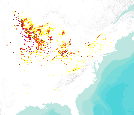This dataset represents actual and predicted suitable habitat for Tilia americana (American basswood, species code 951) in the Eastern United States as measured by importance value based on data obtained from the Forest Inventory and Analysis (FIA) project, current climate conditions, and future climate projections. This summary unit of this dataset is a 20 by 20 kilometer cell. The actual importance value (IV) was calculated based on the number of stems and basal area of a given tree species relative to other tree species on a plot using about 100,000 FIA plots (representing nearly 3 million tree records) in the 37 states within the United States east of the 100th meridian. These importance values were summarized...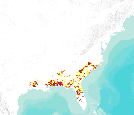This dataset represents actual and predicted suitable habitat for Pinus palustris (longleaf pine, species code 121) in the Eastern United States as measured by importance value based on data obtained from the Forest Inventory and Analysis (FIA) project, current climate conditions, and future climate projections. This summary unit of this dataset is a 20 by 20 kilometer cell. The actual importance value (IV) was calculated based on the number of stems and basal area of a given tree species relative to other tree species on a plot using about 100,000 FIA plots (representing nearly 3 million tree records) in the 37 states within the United States east of the 100th meridian. These importance values were summarized to...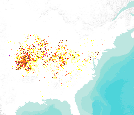This dataset represents actual and predicted suitable habitat for Juglans nigra (black walnut, species code 602) in the Eastern United States as measured by importance value based on data obtained from the Forest Inventory and Analysis (FIA) project, current climate conditions, and future climate projections. This summary unit of this dataset is a 20 by 20 kilometer cell. The actual importance value (IV) was calculated based on the number of stems and basal area of a given tree species relative to other tree species on a plot using about 100,000 FIA plots (representing nearly 3 million tree records) in the 37 states within the United States east of the 100th meridian. These importance values were summarized to 20...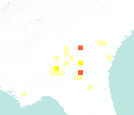This dataset represents actual and predicted suitable habitat for Nyssa ogeche (Ogechee tupelo, species code 692) in the Eastern United States as measured by importance value based on data obtained from the Forest Inventory and Analysis (FIA) project, current climate conditions, and future climate projections. This summary unit of this dataset is a 20 by 20 kilometer cell. The actual importance value (IV) was calculated based on the number of stems and basal area of a given tree species relative to other tree species on a plot using about 100,000 FIA plots (representing nearly 3 million tree records) in the 37 states within the United States east of the 100th meridian. These importance values were summarized to...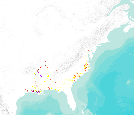This dataset represents actual and predicted suitable habitat for Nyssa aquatica (water tupelo, species code 691) in the Eastern United States as measured by importance value based on data obtained from the Forest Inventory and Analysis (FIA) project, current climate conditions, and future climate projections. This summary unit of this dataset is a 20 by 20 kilometer cell. The actual importance value (IV) was calculated based on the number of stems and basal area of a given tree species relative to other tree species on a plot using about 100,000 FIA plots (representing nearly 3 million tree records) in the 37 states within the United States east of the 100th meridian. These importance values were summarized to...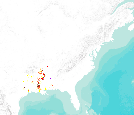This dataset represents actual and predicted suitable habitat for Carya aquatica (water hickory, species code 401) in the Eastern United States as measured by importance value based on data obtained from the Forest Inventory and Analysis (FIA) project, current climate conditions, and future climate projections. This summary unit of this dataset is a 20 by 20 kilometer cell. The actual importance value (IV) was calculated based on the number of stems and basal area of a given tree species relative to other tree species on a plot using about 100,000 FIA plots (representing nearly 3 million tree records) in the 37 states within the United States east of the 100th meridian. These importance values were summarized to...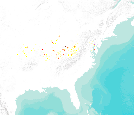This dataset represents actual and predicted suitable habitat for Asimina triloba (pawpaw, species code 367) as measured by importance value based on data obtained from the Forest Inventory and Analysis (FIA) project, current climate conditions, and future climate projections. This summary unit of this dataset is a 20 by 20 kilometer cell. The actual importance value (IV) was calculated based on the number of stems and basal area of a given tree species relative to other tree species on a plot using about 100,000 FIA plots (representing nearly 3 million tree records) in the 37 states within the United States east of the 100th meridian. These importance values were summarized to 20 by 20 kilometer grid cells. Current...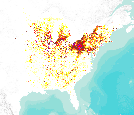This dataset represents actual and predicted suitable habitat for Prunus serotina (black cherry, species code 762) in the Eastern United States as measured by importance value based on data obtained from the Forest Inventory and Analysis (FIA) project, current climate conditions, and future climate projections. This summary unit of this dataset is a 20 by 20 kilometer cell. The actual importance value (IV) was calculated based on the number of stems and basal area of a given tree species relative to other tree species on a plot using about 100,000 FIA plots (representing nearly 3 million tree records) in the 37 states within the United States east of the 100th meridian. These importance values were summarized to...This dataset represents actual and predicted suitable habitat for Quercus durandii (Durand oak, species code 808) in the Eastern United States as measured by importance value based on data obtained from the Forest Inventory and Analysis (FIA) project, current climate conditions, and future climate projections. This summary unit of this dataset is a 20 by 20 kilometer cell. The actual importance value (IV) was calculated based on the number of stems and basal area of a given tree species relative to other tree species on a plot using about 100,000 FIA plots (representing nearly 3 million tree records) in the 37 states within the United States east of the 100th meridian. These importance values were summarized to...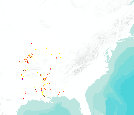This dataset represents actual and predicted suitable habitat for Carya illinoensis (pecan, species code 404) in the Eastern United States as measured by importance value based on data obtained from the Forest Inventory and Analysis (FIA) project, current climate conditions, and future climate projections. This summary unit of this dataset is a 20 by 20 kilometer cell. The actual importance value (IV) was calculated based on the number of stems and basal area of a given tree species relative to other tree species on a plot using about 100,000 FIA plots (representing nearly 3 million tree records) in the 37 states within the United States east of the 100th meridian. These importance values were summarized to 20 by...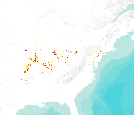This dataset represents actual and predicted suitable habitat for Quercus palustris (pin oak, species code 830) in the Eastern United States as measured by importance value based on data obtained from the Forest Inventory and Analysis (FIA) project, current climate conditions, and future climate projections. This summary unit of this dataset is a 20 by 20 kilometer cell. The actual importance value (IV) was calculated based on the number of stems and basal area of a given tree species relative to other tree species on a plot using about 100,000 FIA plots (representing nearly 3 million tree records) in the 37 states within the United States east of the 100th meridian. These importance values were summarized to 20...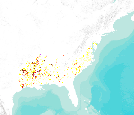This dataset represents actual and predicted suitable habitat for Quercus phellos (willow oak, species code 831) in the Eastern United States as measured by importance value based on data obtained from the Forest Inventory and Analysis (FIA) project, current climate conditions, and future climate projections. This summary unit of this dataset is a 20 by 20 kilometer cell. The actual importance value (IV) was calculated based on the number of stems and basal area of a given tree species relative to other tree species on a plot using about 100,000 FIA plots (representing nearly 3 million tree records) in the 37 states within the United States east of the 100th meridian. These importance values were summarized to 20...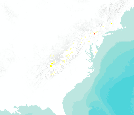This dataset represents actual and predicted suitable habitat for Castanea dentata (American chestnut, species code 421) as measured by importance value based on data obtained from the Forest Inventory and Analysis (FIA) project, current climate conditions, and future climate projections. This summary unit of this dataset is a 20 by 20 kilometer cell. The actual importance value (IV) was calculated based on the number of stems and basal area of a given tree species relative to other tree species on a plot using about 100,000 FIA plots (representing nearly 3 million tree records) in the 37 states within the United States east of the 100th meridian. These importance values were summarized to 20 by 20 kilometer grid...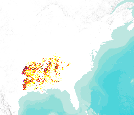This dataset represents actual and predicted suitable habitat for Ulmus alata (winged elm, species code 971) in the Eastern United States as measured by importance value based on data obtained from the Forest Inventory and Analysis (FIA) project, current climate conditions, and future climate projections. This summary unit of this dataset is a 20 by 20 kilometer cell. The actual importance value (IV) was calculated based on the number of stems and basal area of a given tree species relative to other tree species on a plot using about 100,000 FIA plots (representing nearly 3 million tree records) in the 37 states within the United States east of the 100th meridian. These importance values were summarized to 20 by...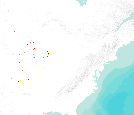This dataset represents actual and predicted suitable habitat for Prunus americana (wild plum, species code 766) in the Eastern United States as measured by importance value based on data obtained from the Forest Inventory and Analysis (FIA) project, current climate conditions, and future climate projections. This summary unit of this dataset is a 20 by 20 kilometer cell. The actual importance value (IV) was calculated based on the number of stems and basal area of a given tree species relative to other tree species on a plot using about 100,000 FIA plots (representing nearly 3 million tree records) in the 37 states within the United States east of the 100th meridian. These importance values were summarized to 20...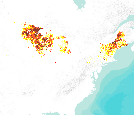This dataset represents actual and predicted suitable habitat for Betula papyrifera (paper birch, species code 375) in the Eastern United States as measured by importance value based on data obtained from the Forest Inventory and Analysis (FIA) project, current climate conditions, and future climate projections. This summary unit of this dataset is a 20 by 20 kilometer cell. The actual importance value (IV) was calculated based on the number of stems and basal area of a given tree species relative to other tree species on a plot using about 100,000 FIA plots (representing nearly 3 million tree records) in the 37 states within the United States east of the 100th meridian. These importance values were summarized to...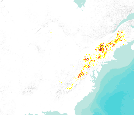This dataset represents actual and predicted suitable habitat for Acer pensylvanicum (striped maple, species code 315) in the Eastern United States as measured by importance value based on data obtained from the Forest Inventory and Analysis (FIA) project, current climate conditions, and future climate projections. This summary unit of this dataset is a 20 by 20 kilometer cell. The actual importance value (IV) was calculated based on the number of stems and basal area of a given tree species relative to other tree species on a plot using about 100,000 FIA plots (representing nearly 3 million tree records) in the 37 states within the United States east of the 100th meridian. These importance values were summarized... 12345...7»